# 978-0134292380 Chapter 6 Part 2

Document Type
Test Prep
Book Title
Fundamentals of Hydraulic Engineering Systems 5th Edition
Authors
A. Osman H. Akan, Ned H. C. Hwang, Robert J. Houghtalen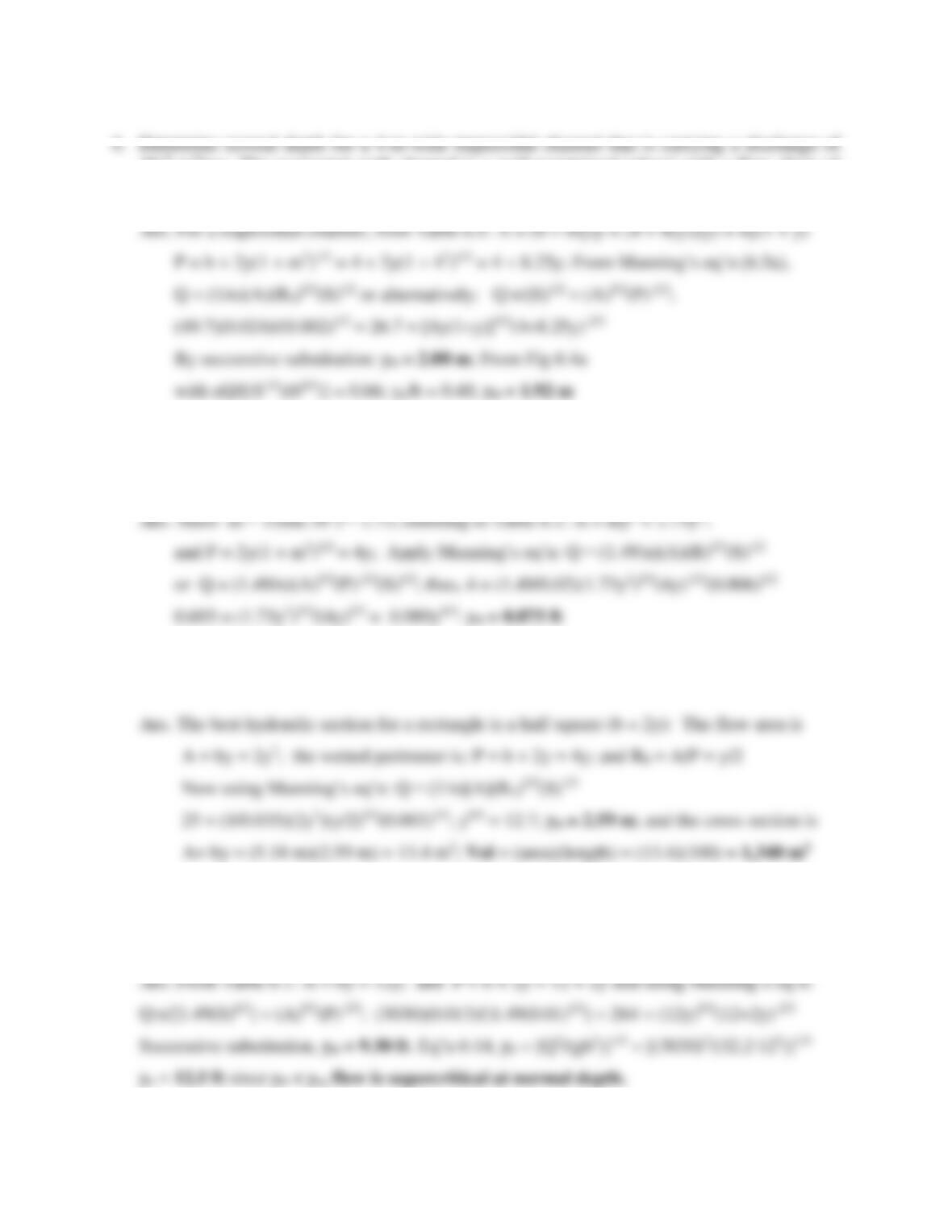61
49.7 m3/sec. The excavated earth channel is a well-maintained (clean) with a flow slope of
0.2% and side slopes of 4:1 (H:V). Solve using successive substitution and Figure 6.4.
5. A grass-lined roadside channel (n = 0.02) is triangular in shape with 30° side slopes and a
bottom slope of 0.006. Determine the normal depth of flow when the discharge is 4 cfs
(ft3/sec). Check your solution using appropriate computer software.
6. Find the best hydraulic (rectangular) channel cut in rock (n = 0.035) to pass 25m3/sec on a
slope of 0.003 m/m. Determine the excavation volume of the 100-m-long channel
7. A 12-ft-wide rectangular channel conveys 3,030 cfs. Determine the normal and critical
depths for the concrete channel (1% slope). Also determine the flow classification (critical,
supercritical, or subcritical) when the channel flows at normal depth.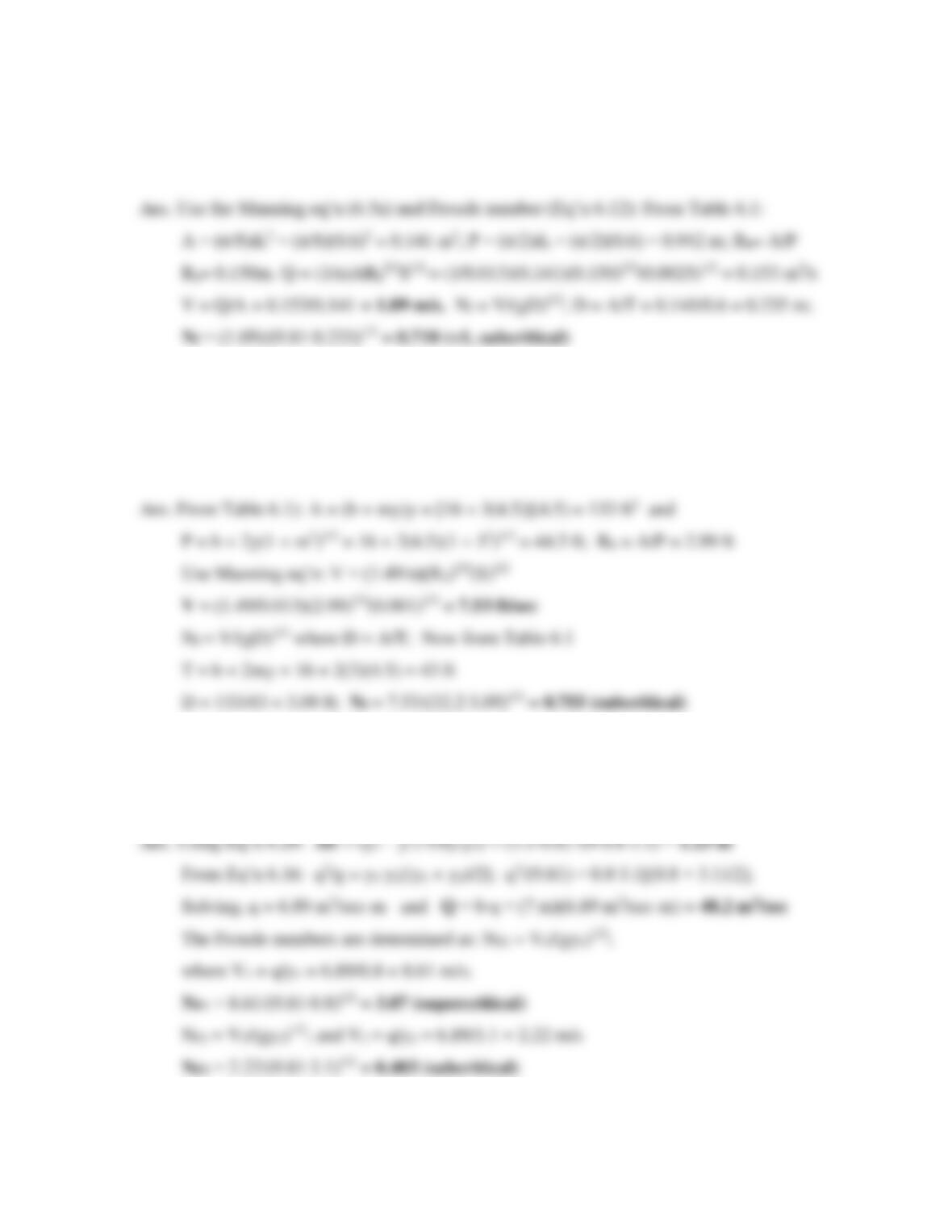62
8. A 60-cm-diameter concrete pipe on a 1:400 slope carries water at a depth of 30 cm.
Determine the flow rate and flow classification (subcritical, critical, or supercritical).
9. Water flows in a long, uniform trapezoidal channel lined with brick (n = 0.013) at a depth of
4.5 feet. The base width of the channel is 16.0 ft., the side slopes are 3:1 (H:V), and the
bottom drops a foot for every 1000 feet of length. Determine the velocity of flow and the
flow classification (subcritical, critical, or supercritical).
10. A hydraulic jump occurs in a rectangular channel that is 7 m wide. The initial depth is 0.8 m
and the sequent depth if 3.1 m. Determine the energy loss and the discharge in the channel.
Also determine the Froude number before and after the jump.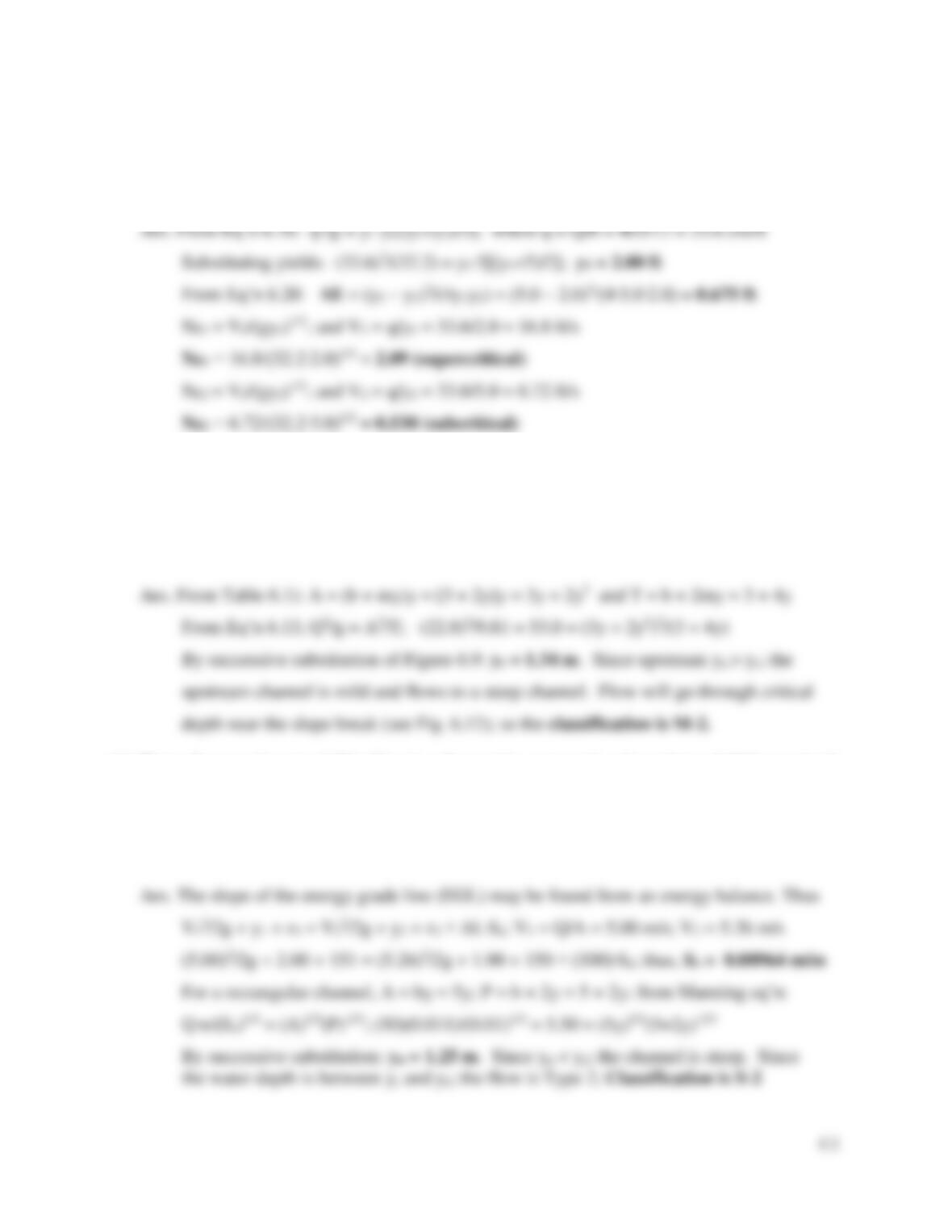63
11. A hydraulic jump occurs in 12-ft wide rectangular channel. If the flow rate is 403 cfs and the
downstream depth is 5 feet, determine the upstream depth, the loss of energy in the jump, and
the Froude number before and after the jump.
12. Water flows at a rate of 22.8 m3/sec in a trapezoidal channel (n = 0.04) with a bottom width
of 3 m, side slopes of 2:1 (H:V), and a bottom slope of 0.005. At a certain location, the
bottom slope increases to 0.022 with no change in channel dimensions. Normal depths are
1.83 m (upstream) and 1.27 m (downstream). Determine the channel and flow classification
(e.g., M-3, S-2, etc.) just upstream of the break in slope. Explain your supporting logic.
13. Water flows at the rate of 50 m3/sec in a 5-m-wide, rectangular channel (n = 0.011) at a depth
of 1.90 m where the bottom elevation is 150 m above MSL. Upstream 100 m, the depth is
2.00 m where the bottom elevation is 151 m above MSL. What is the slope of the energy
grade line in this section of channel? If critical depth is 2.17 m, determine the channel and
flow classification (e.g., M-3, S-2, etc.). (Note: MSL stands for Mean Sea Level, the datum.)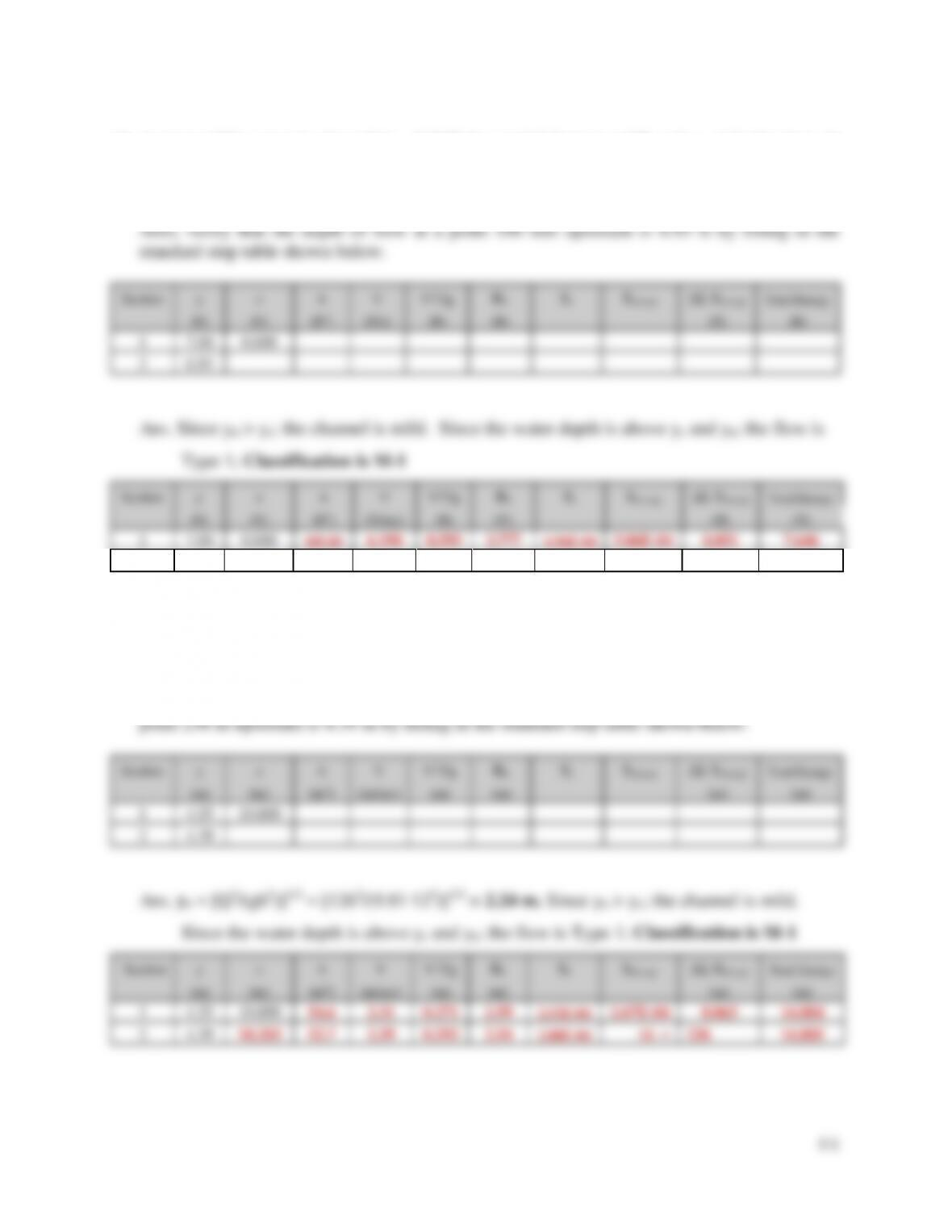14. A trapezoidal, concrete channel (n = 0.013) has an 8-ft bottom width and z = 1.0 side slope. It
discharges 650 ft3/sec on a 0.001 channel slope. The channel is flowing at normal depth (5.85
ft) when it encounters an obstruction. The obstruction causes the depth to rise to 7.00 ft. If
critical depth is 4.80 ft, determine the channel and flow classification (e.g., M-3, S-2, etc.).
105.00
6.190
0.595
3.777
4.96E-04
0.051
7.646
2
6.93
0.100
103.46
6.282
0.613
3.749
5.16E-04
100
7.643
15. Water flowing at normal depth (2.95 m) in a rectangular concrete channel (n = 0.013) that is
12 m wide encounters an obstruction. The water level rises to 4.55 m to flow over the
obstruction. The flow rate is 126 m3/sec and the bottom slope is 0.00086. Determine the
channel and flow classification (e.g., M-3, S-2, etc.). Also, verify that the depth of flow at a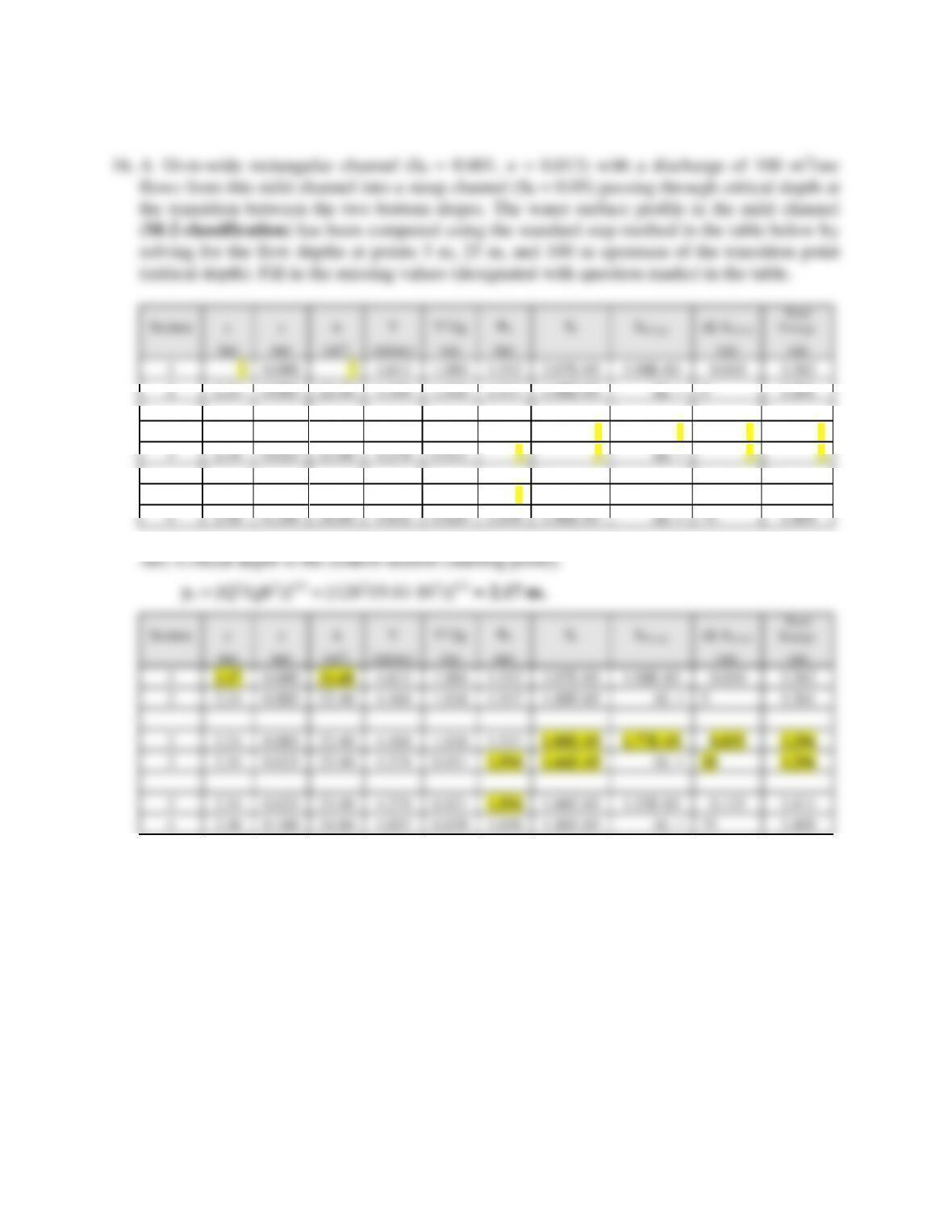2.07E-03
1.98E-03
3.262
2
2.24
22.40
4.464
1.016
1.547
1.88E-03
ΔL =
5
3.261
2
2.24
0.005
22.40
4.464
1.016
1.547
?
?
?
?
3
2.34
23.40
4.274
0.931
?
?
ΔL =
?
?
3
2.34
0.025
23.40
4.274
0.931
?
1.66E-03
1.53E-03
0.115
3.411
4
2.48
24.80
4.032
0.829
1.658
1.40E-03
ΔL =
75
3.409
1.40E-03
ΔL =
75
3.409
17. Flow enters a long, rectangular flume at its upstream end from under a sluice gate. The flume
has a bottom width of 3 ft, n = 0.013, and a bottom slope of So = 0.02. The flow depth at the
entrance is 1.30 ft and the discharge is 30 cfs. The normal and critical depths have already
been determined as yn = 0.91 ft and yc = 1.46 ft.
a) Determine the channel and flow classification in the flume (e.g., M-2, S-1, etc.) and
b) Will depths of 0.80 ft and 0.60 ft ft occur in this channel? If your answer is YES calculate
how far downstream from the sluice gate. If your answer is NO explain why.
c) Will depths of 1.20 ft and 1.10 ft occur in this channel? If your answer is YES calculate
how far downstream from the sluice gate. If your answer is NO explain.(20 points).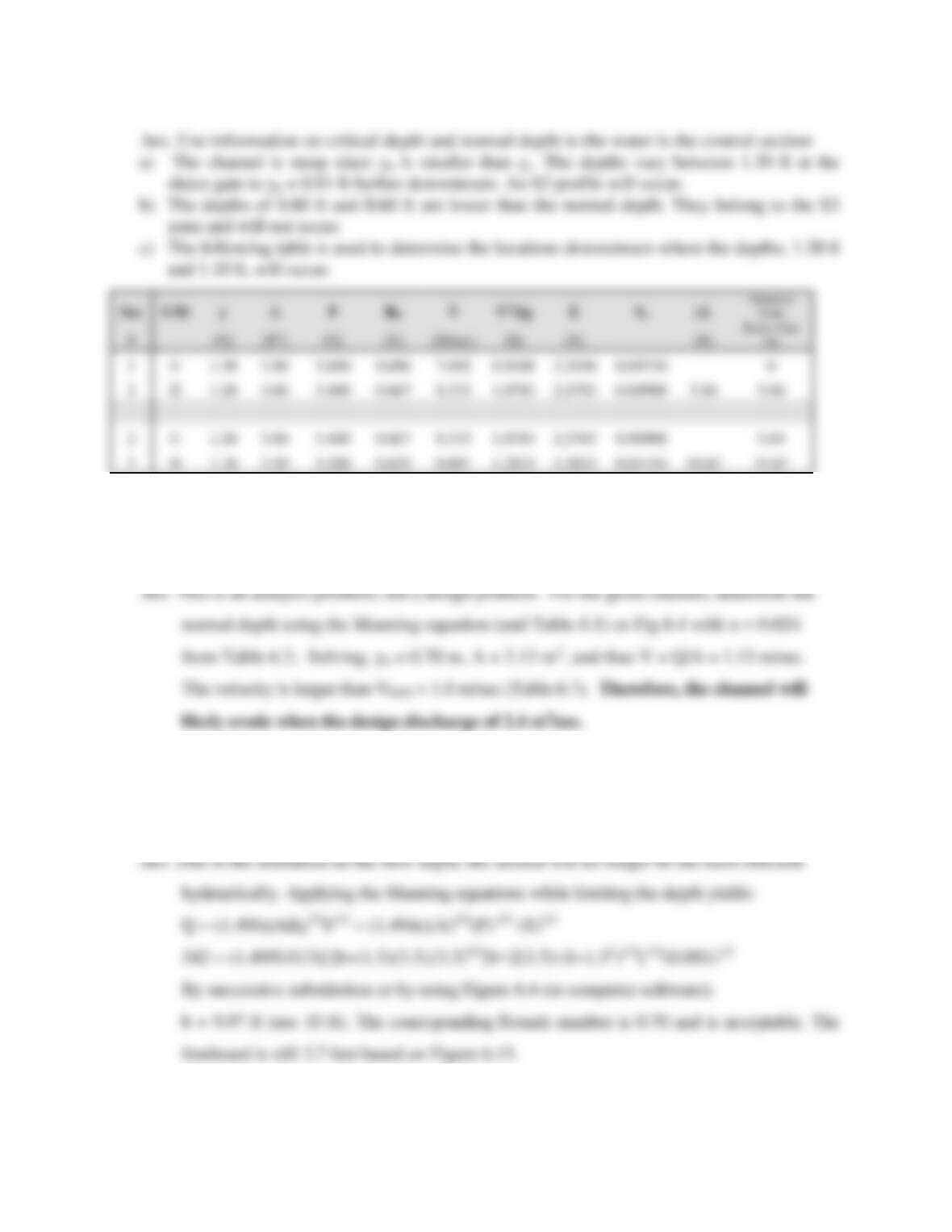66
10.83
15.87
18. An irrigation channel is to be constructed in silt clay (earth excavation, clean) with side slopes
of m = 1.5, a bottom slope of S0 = 0.002 m/m, and a bottom width of b = 2.0 m. Can this
channel convey 2.4 m3/sec without being eroded?
19. The best hydraulic section for a channel that passes 342 cfs (n = 0.013, m = 1.5, So = 0.001)
yields b = 3.0 ft and y = 4.95 ft. (prior to freeboard). However, the depth is limited to 3.5 ft due
to a high water table at the site. Resize the channel section considering the depth limitation.

## Trusted by Thousands ofStudents

Here are what students say about us.